# The specific heat capacity of solid lead is 0.159J/g°C, the molar enthalpy of fusion is 5.0kJ/mol, and the melting point of lead is 328°C

The specific heat capacity of solid lead is 0.159J/g°C, the molar enthalpy of fusion is 5.0kJ/mol, and the melting point of lead is 328°C. Calculate the total energy required to change 100g of lead at 25°C to molten lead at it's melting point.

I will be happy to critique your work or thinking. See the other posts in this series for hints.

Fair enough, the least I can do is try it. Basically I will find the heat required to bring the lead to it's melting point using q = mc(delta)t.
So, q = (100g)(0.159/J/g°C)(328°C-25°C)....
q = 4.8x10^3 J.

After finding that out I need to find the energy required to actually melt the lead and change it's form from solid to a liquid. I would use q = n(delta)H.

n = the ammount of moles per the 100g given. So 100g / 207.2g/mol = 0.483mol.

q = (0.483mol)(5.0kJ/mol) = 2.4kJ.
----------------------------------------
So I have some numbers, and I assume that I add the two together. If I do infact have to do this, which unit (kJ or J) should I express it in?

IT is correct. It does not matter which unit 2.4kJ is 2400 joules. The common practice in scientific notation would be to write it as 2.4kJ

Thanks for the help bobpursley :)

ok i just wanted to know the name of the course/class using graphics for the change over solids to gases using the (m.c.delta T)formula and what grade it's taught :P thanks....

We need at least 10 more requests to produce the answer.

0 / 10 have requested this problem solution

The more requests, the faster the answer.

All students who have requested the answer will be notified once they are available.

#### Earn Coin

Coins can be redeemed for fabulous gifts.

Similar Homework Help Questions
• ### The following information is given for lead at latm: AH yap(1740 °C) -178 kJ/mol AH fus(328...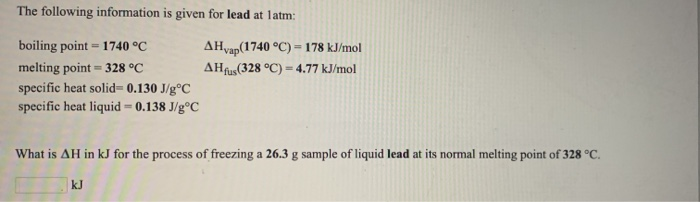The following information is given for lead at latm: AH yap(1740 °C) -178 kJ/mol AH fus(328 °C) = 4.77 kJ/mol boiling point = 1740 °C melting point = 328 °C specific heat solid= 0.130 J/gºC specific heat liquid = 0.138 J/gºC What is AH in kJ for the process of freezing a 26.3 g sample of liquid lead at its normal melting point of 328 °C. The following information is given for silver at latm: AHvap(2212 °C) = 254 kJ/mol...

• ### The change in enthalpy when 1 mol of ice is melted at 273K is 6008 J Heat Capacity of liquid water, Cp_{L} = 75.44 J/mol K Heat Capacity of solid water, Cp_{S} = 38J/mol K Enthalpy chage of melting at...

The change in enthalpy when 1 mol of ice is melted at 273K is 6008 J Heat Capacity of liquid water, Cp_{L} = 75.44 J/mol K Heat Capacity of solid water, Cp_{S} = 38J/mol K Enthalpy chage of melting at 273K, \Delta H_{273}=6008 J Calculate the standard enthalpy of fusion for ice. Calculate the heat released when 100 g of water supercooled at 250K solidify Initial T=25°C=298K Thanks

• ### A piece of solid lead weighing 32.6 g at a temperature of 311 °C is placed...

A piece of solid lead weighing 32.6 g at a temperature of 311 °C is placed in 326 g of liquid lead at a temperature of 367 °C. After a while, the solid melts and a completely liquid sample remains. Calculate the temperature after thermal equilibrium is reached, assuming no heat loss to the surroundings. The enthalpy of fusion of solid lead is ΔHfus = 4.77 kJ/mol at its melting point of 328 °C, and the molar heat capacities for...

• ### A piece of solid lead weighing 34.2 g at a temperature of 315 °C is placed...

A piece of solid lead weighing 34.2 g at a temperature of 315 °C is placed in 342 g of liquid lead at a temperature of 376 °C. After a while, the solid melts and a completely liquid sample remains. Calculate the temperature after thermal equilibrium is reached, assuming no heat loss to the surroundings. The enthalpy of fusion of solid lead is ΔHfus = 4.77 kJ/mol at its melting point of 328 °C, and the molar heat capacities for...

• ### LEAD IS A SOFT, DENSE METAL WITH A SPECIFIC HEAT OF 0.028KCAL/KGC, A MELTING POINT OF 328.0C, AND A HEAT FUSION OF5.5KCAL/KG

LEAD IS A SOFT, DENSE METAL WITH A SPECIFIC HEAT OF 0.028KCAL/KGC, A MELTING POINT OF 328.0C, AND A HEAT FUSION OF5.5KCAL/KG. HOW MUCH HEAT MUST BE PROVIDED TO MELT A 250.0KG SAMPLE OF LEAD WITH A TEMPERATURE OF 20.0C? Figure the heat to heat if from 20C to the melting point (Q=mc deltaT) Add the heat needed to melt it at the melting point (Q=heatfusion*mass) I CAME UP WITH 1,640KCAL I AM RIGHT? No. Break it down in to...

• ### 14. For bismuth, Bi, the heat of fusion at its normal melting point of 271 °C...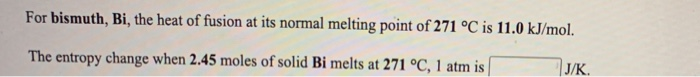14. For bismuth, Bi, the heat of fusion at its normal melting point of 271 °C is 11.0 kJ/mol. The entropy change when 2.45 moles of solid Bi melts at 271 °C, 1 atm is J/K. For magnesium, Mg, the heat of fusion at its normal melting point of 649 °C is 9.0 kJ/mol. The entropy change when 1.72 moles of liquid Mg freezes at 649 °C, 1 atm is J/K

• ### For ethanol, C2H5OH, the heat of fusion at its normal melting point of -115 °C is...

For ethanol, C2H5OH, the heat of fusion at its normal melting point of -115 °C is 5.0 kJ/mol. The entropy change when 1.84 moles of solid C2H5OH melts at -115 °C, 1 atm is J/K.

• ### For copper, Cu, the heat of fusion at its normal melting point of 1083 °C is...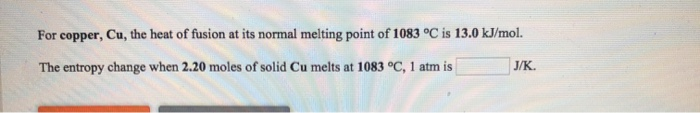For copper, Cu, the heat of fusion at its normal melting point of 1083 °C is 13.0 kJ/mol. The entropy change when 2.20 moles of solid Cu melts at 1083 °C, 1 atm is J/K

• ### 6. The melting point of CaTiSiOs is 1400°C and the heat of fusion at the normal melting point is 123 700 J mol-1. Calculate the heat of fusion at 1300°C. Cnsolid = 177.4 + 23.2 x 10', 40.3 x...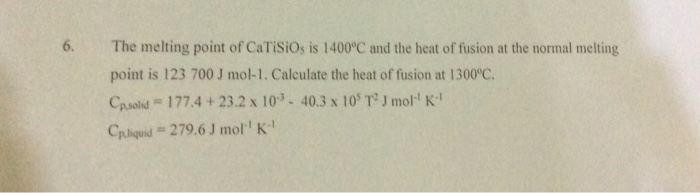6. The melting point of CaTiSiOs is 1400°C and the heat of fusion at the normal melting point is 123 700 J mol-1. Calculate the heat of fusion at 1300°C. Cnsolid = 177.4 + 23.2 x 10', 40.3 x 105 T2 J mor' K" Cphquid # 279.6 J mol'' K" 6. The melting point of CaTiSiOs is 1400°C and the heat of fusion at the normal melting point is 123 700 J mol-1. Calculate the heat of fusion at 1300°C....

• ### The enthalpy of fusion of cadmium at its normal melting point of 321 °C is 6.11...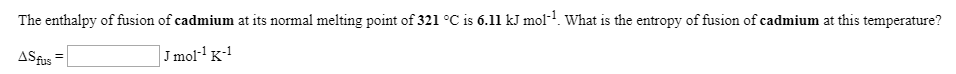The enthalpy of fusion of cadmium at its normal melting point of 321 °C is 6.11 kJ mol? What is the entropy of fusion of cadmium at this temperature? ASfus = J mol-K-1 The molar enthalpy of fusion of solid cadmium is 6.11 kJ mol-1, and the molar entropy of fusion is 10.3 JK+mol-1. (a) Calculate the Gibbs free energy change for the melting of 1.00 mol of cadmium at 622 K. (b) Calculate the Gibbs free energy change for...